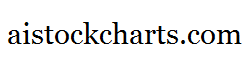Members Area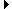Sign Up | Log In
 Free Level 2 QuotesStock AnalysisCorrelation Trading PlatformCorrelationsMembership Benefits

P4RANGE
 Lookup a different indicator.

 Indicator Usage Description Example p4range p4range[n] OPERATOR (INDICATOR or POSITIVE NUMBER) , where n is a positive integer >= 0. Returns the percentage change from the lowest price among day n, day n-1, day n-2 and day n-3 to the highest price among day n, day n-1, day n-2 and day n-3 where n=0 is the last trading day, n=1 is the previous day, etc. (p4range < 3)

Introduction:

The 'p4range' indicator is used in indicator formula construction to narrow the stock pre-screener results to include only those stocks that have a percentage change from the lowest to highest price of 4 consecutive days that are restricted to the parameters set for the p4range indicator.

Example:

The chart of Intel (INTC) below highlights days where the percentage change from the lowest to highest stock price of 4 consecutive days was less than 3%.

 Chart of Intel (INTC) highlighting days where the percentage change from the lowest to highest price of 4 consecutive days was less than 3%.

INDICATORS IN OUR DATABASE THAT INCLUDE THE P4RANGE INDICATOR...

Click here to view all indicators that include the indicator 'p4range' (sorted by AISCORE)

 (p4range < 3)AISCORE: 2001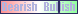Indicator ID: 060131092709(p4range < 2)AISCORE: 1525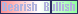Indicator ID: 060131092939(close < sma) and (close > sma) and (vol > 1.1 * vol) and (p4range < 10)AISCORE: 1553Indicator ID: 060519103520(p4range > 1) and (p2change > 1) and (p1range > 4)AISCORE: 916Indicator ID: 060811174707(imi < 71) and (p4range > 12) and (p4change < -5)AISCORE: 3010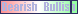Indicator ID: 060811180921 More ->